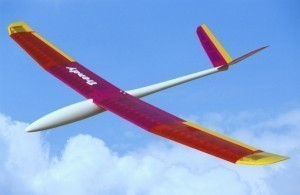# Glider Dimensions

There are numerous types of gliders out there, each with their own particular specs.These models should serve as an example of their diversity.

### Glider Dimensions: Marsden Gemini

The span is 18.5 m / 60.75 ft and the area is 11.52 sq. m / 124 sq ft. The aspect ratio is 29.8 and the empty weight is 356 kg. / 785 lb. Its payload is 220 kg. / 455 lb and the gross weight is 576 kg. / 1,240 lb.

The wing loading is 50.0 kg. / sq. m. / 10.0 lb. / sq. ft and the L / D max is 40 119 kph /64 kt / 74 mph. There are two seats and this glider was developed in Canada. This was first flown in 1973. Except for the GFRP forward fuselage, the whole structure is composed of metal.

### Glider Dimensions: Caproni A-21

The span is 20.38 m / 66.9 ft and the aspect ratio is 25.65. The area is 16.19 sq. m / 174.3 sq ft and the empty weight is 436 kg. / 961 lb. (529 kg / 1,167 lb. The gross weight is 644 kg. / 1420 lb. (810 kg / 1,786 lb and the payload is 208 kg. / 459 lb. (281 kg / 619 lb.).

The wing loading is 39.8 kg. / sq. m / 8.16 lb. / sq. ft and the engine is A-21 SJ. The L/D max is 43 105 kph/ 56 kt / 65 mph. This glider was designed by Carlo Ferrarin and Livio Sonzio in Italy.

There are two seats and 100 models exist. The minimum sink is 0.61 m/s / 2.0 fps / 1.18 kt and the structure is composed of metal and fiberglass.

### Glider Dimensions: Explorer PG-1 Aque Glider

The span is 4.87 m / 16 ft and the empty weight is 83 kg. / 180 lb. The area is 14.28 sq. m. / 95 sq ft. The aspect ratio is 5 and the payload is 100 kg. / 220 lb. The wing loading is 20.61 kg. / sq m. / 4.5 lb. / sq. ft and the gross weight is 182 kg / 400 lb.

The glider was designed by Bill Skliar and made in the United States. This glider is a biplane and first flew the skies in 1959. The components are made of fabric and wood.

### Glider Dimensions: Mechta AC-4

Its aspect ratio is 20.5 and the span 12.60 m / 41.3 ft. The area is 7.7 sq. m. / 82.9 sq. ft and the empty weight is 130 kg. / 286 lb. The payload is 120 kg. / 265 lb and the wing loading is 32.46 kg. / sq. m. / 6.66 lb. /sq. ft. The gross weight is 250 kg. / 551 lb.

The L / D max is 31 at 83 kph / 45 kt / 52 mph. The minimum sink is 0.66 m/s / 2.16 fps / 1.28 kt. This was designed in Russia by Vladimir Federov. It has one seat and 30 have been built.

Glider dimensions are subject to change. As models improve, their specs may be altered.